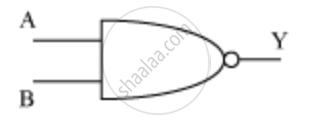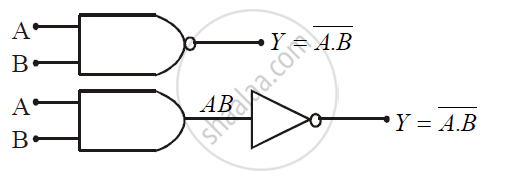# Give the truth table and circuit symbol for NAND gate - Physics (Theory)

Give the truth table and circuit symbol for NAND gate

What is a NAND gate? Write its truth table.

#### Solution 1

The output is the inverted version of the output of AND gate. The symbol for NAND gate is given belowTruth table

 I O O/P A B Y 0 0 1 0 1 1 1 0 1 1 1 0

#### Solution 2

NAND gate is the combination of AND gate and NOT gate i.e. NAND gate is nothing but it is AND gate with an inverted output.

The Logical statement of NAND gate is if input A is true and B is true then the output if false otherwise
true.

The Boolean equation of NAND gate is Y = bar(A.B)

 Input Output A B Y = bar(A.B) 0 0 1 0 1 1 1 0 1 1 1 0Concept: Digital Electronics and Logic Gates
Is there an error in this question or solution?